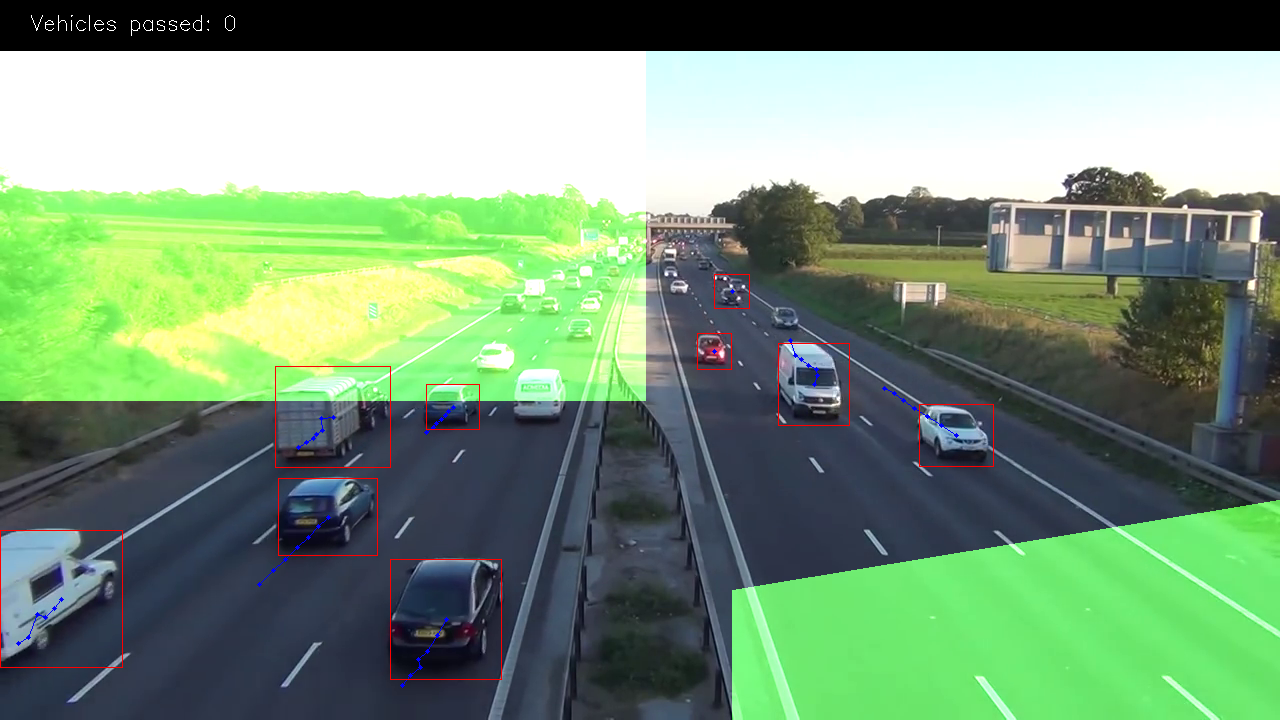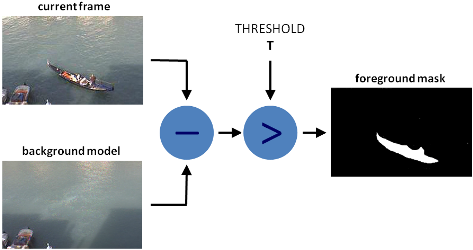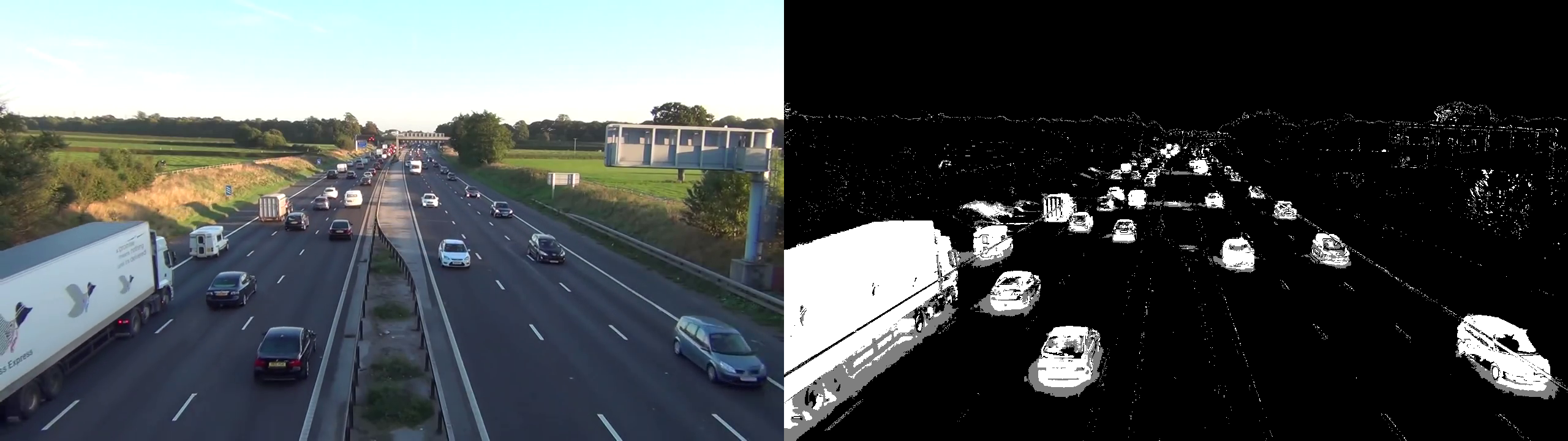Go to Practical Course

# Making Road Traffic Counting App

Great tutorial to understand strong and week spots on classical Computer VisionToday we will learn how to count road traffic based on computer vision and without heavy deep learning algorithms.
For this tutorial, we will use only Python and OpenCV with the pretty simple idea of motion detection with help of background subtraction algorithm.

Here is our plan:

• Understand the main idea of background subtraction algorithms that used for foreground detection.
• OpenCV image filters.
• Object detection by contours.
• Building processing pipeline for further data manipulation.

## Background subtraction algorithmsThere are many different algorithms for background subtraction, but the main idea of them is very simple.
Let’s assume that you have a video of your room, and on some of the frames of this video there is no humans & pets, so basically it’s static, let’s call it background_layer. So to get objects that are moving on the video we just need to:

foreground_objects = current_frame - background_layer

But in some cases, we cant get static frame because lighting can change, or some objects will be moved by someone, or always exist movement, etc. In such cases we are saving some number of frames and trying to figure out which of the pixels are the same for most of them, then this pixels becoming part of background_layer. Difference generally in how we get this background_layer and additional filtering that we use to make selection more accurate.
In this lesson, we will use MOG algorithm for background subtraction and after processing, it looks like thisdef train_bg_subtractor(inst, cap, num=500):
'''
BG substractor need process some amount
of frames to start giving result
'''
print ('Training BG Subtractor...')
i = 0
for frame in cap:
inst.apply(frame, None, 0.001)
i += 1
if i >= num:
return cap

bg_subtractor = cv2.createBackgroundSubtractorMOG2(

# Set up image source

# skipping 500 frames to train bg subtractor
train_bg_subtractor(bg_subtractor, cap, num=500)

frame = next(cap)

plt.figure(figsize=(12,12))
plt.show()As you can see there is some noise on the foreground mask which we will try to remove with some standard filtering technic.

## Filtering image

For our case we will need this filters: Threshold, Erode, Dilate, Opening, Closing Please go by links and read about each of them and look how they work (to not make copy/paste)

So now we will use them to remove some noise on foreground mask. First, we will use Closing to remove gaps in areas, then Opening to remove 1–2 px points, and after that dilation to make object bolder.


'''
This filters are hand-picked just based on visual tests
'''

kernel = cv2.getStructuringElement(cv2.MORPH_ELLIPSE, (2, 2))

# Fill any small holes
closing = cv2.morphologyEx(img, cv2.MORPH_CLOSE, kernel)
# Remove noise
opening = cv2.morphologyEx(closing, cv2.MORPH_OPEN, kernel)

# Dilate to merge adjacent blobs
dilation = cv2.dilate(opening, kernel, iterations=2)

return dilation

bg_subtractor = cv2.createBackgroundSubtractorMOG2(

# Set up image source

# skipping 500 frames to train bg subtractor
train_bg_subtractor(bg_subtractor, cap, num=500)

frame = next(cap)

plt.figure(figsize=(12,12))
plt.show()## Object detection by contours

For this purpose we will use the standard cv2.findContours method with params:


cv2.CV_RETR_EXTERNAL — get only outer contours.
cv2.CV_CHAIN_APPROX_TC89_L1 - use Teh-Chin chain approximation algorithm (faster)


def get_centroid(x, y, w, h):
x1 = int(w / 2)
y1 = int(h / 2)

cx = x + x1
cy = y + y1

return (cx, cy)

class ContourDetection:
'''
Detecting moving objects.

Purpose of this processor is to subtrac background, get moving objects
and detect them with a cv2.findContours method, and then filter off-by
width and height.

bg_subtractor - background subtractor isinstance.
min_contour_width - min bounding rectangle width.
min_contour_height - min bounding rectangle height.
save_image - if True will save detected objects mask to file.
image_dir - where to save images(must exist).
'''

def __init__(self, bg_subtractor, min_contour_width=35,
min_contour_height=35, save_image=False,
image_dir='images'):
super(ContourDetection, self).__init__()

self.bg_subtractor = bg_subtractor
self.min_contour_width = min_contour_width
self.min_contour_height = min_contour_height
self.save_image = save_image
self.image_dir = image_dir

'''
This filters are hand-picked just based on visual tests
'''

kernel = cv2.getStructuringElement(cv2.MORPH_ELLIPSE, (2, 2))

# Fill any small holes
closing = cv2.morphologyEx(img, cv2.MORPH_CLOSE, kernel)
# Remove noise
opening = cv2.morphologyEx(closing, cv2.MORPH_OPEN, kernel)

# Dilate to merge adjacent blobs
dilation = cv2.dilate(opening, kernel, iterations=2)

return dilation

matches = []

# finding external contours
contours, hierarchy = cv2.findContours(

for (i, contour) in enumerate(contours):
(x, y, w, h) = cv2.boundingRect(contour)
# On the exit, we add some filtering by height, width and add centroid.
contour_valid = (w >= self.min_contour_width) and (
h >= self.min_contour_height)

if not contour_valid:
continue

centroid = get_centroid(x, y, w, h)

matches.append(((x, y, w, h), centroid))

return matches

def __call__(self, frame):
frame = frame.copy()

# just thresholding values

cd = ContourDetection(bg_subtractor)

bg_subtractor = cv2.createBackgroundSubtractorMOG2(

# Set up image source

# skipping 500 frames to train bg subtractor
train_bg_subtractor(bg_subtractor, cap, num=500)

frame = next(cap)
objects = cd(frame)

print('Getting list of [((x,y,w,h), (xc,yc)), ...]')
print(objects)


## Building processing pipeline

You must understand that in ML and CV there is no one magic algorithm that making altogether, even if we imagine that such algorithm exists, we still wouldn’t use it because it would be not effective at scale. For example a few years ago Netflix created competition with the prize 3 million dollars for the best movie recommendation algorithm. And one of the team created such, problem was that it just couldn’t work at scale and thus was useless for the company. But still, Netflix paid 1 million to them :)

So now we will build simple processing pipeline, it not for scale just for convenient but the idea the same.


class PipelineRunner(object):
'''
Very simple pipline.

Just run passed processors in order with passing context from one to
another.

You can also set log level for processors.
'''

def __init__(self, pipeline=None, log_level=logging.INFO):
self.pipeline = pipeline or []
self.context = {}
self.log = logging.getLogger(self.__class__.__name__)
self.log.setLevel(log_level)
self.log_level = log_level
self.set_log_level()

def set_context(self, data):
self.context = data

if not isinstance(processor, PipelineProcessor):
raise Exception(
'Processor should be an isinstance of PipelineProcessor.')
processor.log.setLevel(self.log_level)
self.pipeline.append(processor)

def remove(self, name):
for i, p in enumerate(self.pipeline):
if p.__class__.__name__ == name:
del self.pipeline[i]
return True
return False

def set_log_level(self):
for p in self.pipeline:
p.log.setLevel(self.log_level)

def run(self):
for p in self.pipeline:
self.context = p(self.context)

self.log.debug("Frame #%d processed.", self.context['frame_number'])

return self.context

class PipelineProcessor(object):
'''
Base class for processors.
'''

def __init__(self):
self.log = logging.getLogger(self.__class__.__name__)


As input constructor will take a list of processors that will be run in order. Each processor making part of the job. We already have Countour Detection class, just need slightly udate it to use context


def save_frame(frame, file_name, flip=True):
# flip BGR to RGB
if flip:
cv2.imwrite(file_name, np.flip(frame, 2))
else:
cv2.imwrite(file_name, frame)

class ContourDetection(PipelineProcessor):
'''
Detecting moving objects.

Purpose of this processor is to subtrac background, get moving objects
and detect them with a cv2.findContours method, and then filter off-by
width and height.

bg_subtractor - background subtractor isinstance.
min_contour_width - min bounding rectangle width.
min_contour_height - min bounding rectangle height.
save_image - if True will save detected objects mask to file.
image_dir - where to save images(must exist).
'''

def __init__(self, bg_subtractor, min_contour_width=35,
min_contour_height=35, save_image=False,
image_dir='images'):
super(ContourDetection, self).__init__()

self.bg_subtractor = bg_subtractor
self.min_contour_width = min_contour_width
self.min_contour_height = min_contour_height
self.save_image = save_image
self.image_dir = image_dir

'''
This filters are hand-picked just based on visual tests
'''

kernel = cv2.getStructuringElement(cv2.MORPH_ELLIPSE, (2, 2))

# Fill any small holes
closing = cv2.morphologyEx(img, cv2.MORPH_CLOSE, kernel)
# Remove noise
opening = cv2.morphologyEx(closing, cv2.MORPH_OPEN, kernel)

# Dilate to merge adjacent blobs
dilation = cv2.dilate(opening, kernel, iterations=2)

return dilation

matches = []

# finding external contours
contours, hierarchy = cv2.findContours(

for (i, contour) in enumerate(contours):
(x, y, w, h) = cv2.boundingRect(contour)
contour_valid = (w >= self.min_contour_width) and (
h >= self.min_contour_height)

if not contour_valid:
continue

centroid = get_centroid(x, y, w, h)

matches.append(((x, y, w, h), centroid))

return matches

def __call__(self, context):
frame = context['frame'].copy()
frame_number = context['frame_number']

# just thresholding values

if self.save_image:

return context



Now let’s create a processor that will link detected objects on different frames and will create paths, and also will count vehicles that got to the exit zone.


def distance(x, y, type='euclidian', x_weight=1.0, y_weight=1.0):
if type == 'euclidian':
return (math.sqrt(float((x - y)**2)
/ x_weight + float((x - y)**2) / y_weight))

class VehicleCounter(PipelineProcessor):
'''
Counting vehicles that entered in exit zone.

Purpose of this class based on detected object and local cache create
objects pathes and count that entered in exit zone defined by exit masks.

path_size - max number of points in a path.
max_dst - max distance between two points.
'''

x_weight=1.0, y_weight=1.0):
super(VehicleCounter, self).__init__()

self.vehicle_count = 0
self.path_size = path_size
self.pathes = []
self.max_dst = max_dst
self.x_weight = x_weight
self.y_weight = y_weight

def check_exit(self, point):
try:
return True
except:
return True
return False

def __call__(self, context):
objects = context['objects']
context['pathes'] = self.pathes
context['vehicle_count'] = self.vehicle_count
if not objects:
return context

points = np.array(objects)[:, 0:2]
points = points.tolist()

# add new points if pathes is empty
if not self.pathes:
for match in points:
self.pathes.append([match])

else:
# LINE 55 ##################
# link new points with old pathes based on minimum distance between
# points
new_pathes = []

for path in self.pathes:
_min = 999999
_match = None
for p in points:
if len(path) == 1:
# distance from last point to current
d = distance(p, path[-1])
else:
# based on 2 prev points predict next point and calculate
# distance from predicted next point to current
xn = 2 * path[-1] - path[-2]
yn = 2 * path[-1] - path[-2]
d = distance(
p, (xn, yn),
x_weight=self.x_weight,
y_weight=self.y_weight
)

if d < _min:
_min = d
_match = p

if _match and _min <= self.max_dst:
points.remove(_match)
path.append(_match)
new_pathes.append(path)

# do not drop path if current frame has no matches
if _match is None:
new_pathes.append(path)

self.pathes = new_pathes

if len(points):
for p in points:
if self.check_exit(p):
continue
self.pathes.append([p])

# LINE 101 ##################
# save only last N points in path
for i, _ in enumerate(self.pathes):
self.pathes[i] = self.pathes[i][self.path_size * -1:]

# count vehicles and drop counted pathes:
new_pathes = []
for i, path in enumerate(self.pathes):
d = path[-2:]

if (
# need at list two points to count
len(d) >= 2 and
# prev point not in exit zone
not self.check_exit(d) and
# current point in exit zone
self.check_exit(d) and
# path len is bigger then min
self.path_size <= len(path)
):
self.vehicle_count += 1
else:
for p in path:
if self.check_exit(p):
break
new_pathes.append(path)

self.pathes = new_pathes

context['pathes'] = self.pathes
context['objects'] = objects
context['vehicle_count'] = self.vehicle_count

self.log.debug('#VEHICLES FOUND: %s' % self.vehicle_count)

return context


This class a bit complicated so let’s walk through it by parts.This green mask on the image is exit zone, is where we counting our vehicles. For example, we will count only paths that have length more than 3 points(to remove some noise) and the 4th in the green zone. We use masks cause it’s many operation effective and simpler than using vector algorithms.

Just use “binary and” operation to check that point in the area, and that’s all.


EXIT_PTS = np.array([
[[732, 720], [732, 590], [1280, 500], [1280, 720]],
[[0, 400], [645, 400], [645, 0], [0, 0]]
])
SHAPE = (720,1280)
base = np.zeros(SHAPE + (3,), dtype='uint8')
exit_mask = cv2.fillPoly(base, EXIT_PTS, (255, 255, 255))[:, :, 0]

plt.imshow(base)
plt.show()


Now let’s link points in paths at line '# LINE 55 ##################'

On first frame. we just add all points as new paths.

Next if len(path) == 1, for each path in the cache we are trying to find the point(centroid) from newly detected objects which will have the smallest Euclidean distance to the last point of the path.

If len(path) > 1, then with the last two points in the path we are predicting new point on the same line, and finding min distance between it and the current point.

The point with minimal distance added to the end of the current path and removed from the list.

If some points left after this we add them as new paths.

And also we limit the number of points in the path at line '# LINE 101 ##################'

Now we will try to count vehicles that entering in the exit zone. To do this we just take 2 last points in the path and checking that last of them in exit zone, and previous not, and also checking that len(path) should be bigger than limit. The part after else is preventing of back-linking new points to the points in exit zone.

And the last two processor is CSV writer to create report CSV file, and visualization for debugging and nice pictures/videos.


class CsvWriter(PipelineProcessor):

def __init__(self, path, name, start_time=0, fps=15):
super(CsvWriter, self).__init__()

self.fp = open(os.path.join(path, name), 'w')
self.writer = csv.DictWriter(self.fp, fieldnames=['time', 'vehicles'])
self.start_time = start_time
self.fps = fps
self.path = path
self.name = name
self.prev = None

def __call__(self, context):
frame_number = context['frame_number']
count = _count = context['vehicle_count']

if self.prev:
_count = count - self.prev

time = ((self.start_time + int(frame_number / self.fps)) * 100
+ int(100.0 / self.fps) * (frame_number % self.fps))
self.writer.writerow({'time': time, 'vehicles': _count})
self.prev = count

return context

BOUNDING_BOX_COLOUR = (255, 192, 0)
CENTROID_COLOUR = (255, 192, 0)
CAR_COLOURS = [(255, 192, 0)]
EXIT_COLOR = (66, 183, 42)

class Visualizer(PipelineProcessor):

def __init__(self, save_image=True, image_dir='images'):
super(Visualizer, self).__init__()

self.save_image = save_image
self.image_dir = image_dir

return True
return False

def draw_pathes(self, img, pathes):
if not img.any():
return

for i, path in enumerate(pathes):
path = np.array(path)[:, 1].tolist()
for point in path:
cv2.circle(img, point, 2, CAR_COLOURS, -1)
cv2.polylines(img, [np.int32(path)], False, CAR_COLOURS, 1)

return img

for (i, match) in enumerate(pathes):

contour, centroid = match[-1][:2]
continue

x, y, w, h = contour

cv2.rectangle(img, (x, y), (x + w - 1, y + h - 1),
BOUNDING_BOX_COLOUR, 1)
cv2.circle(img, centroid, 2, CENTROID_COLOUR, -1)

return img

_img = np.zeros(img.shape, img.dtype)
_img[:, :] = EXIT_COLOR

# drawing top block with counts
cv2.rectangle(img, (0, 0), (img.shape, 50), (0, 0, 0), cv2.FILLED)
cv2.putText(img, ("Vehicles passed: {total} ".format(total=vehicle_count)), (30, 30),
cv2.FONT_HERSHEY_SIMPLEX, 0.7, (255, 255, 255), 1)
return img

def __call__(self, context):
frame = context['frame'].copy()
frame = np.ascontiguousarray(np.flip(frame, 2))
frame_number = context['frame_number']
pathes = context['pathes']
vehicle_count = context['vehicle_count']

frame = self.draw_pathes(frame, pathes)
if self.save_image:
save_frame(frame, self.image_dir +
"/processed_%04d.png" % frame_number)

context['frame'] = frame
return context



CSV writer is saving data by time, cause we need it for further analytics. So i use this formula to add additional frame timing to the unixtimestamp:


time = ((self.start_time + int(frame_number / self.fps)) * 100
+ int(100.0 / self.fps) * (frame_number % self.fps))


so with start time=1 000 000 000 and fps=10 i will get results like this
frame 1 = 1 000 000 000 010
frame 1 = 1 000 000 000 020
…


Then after you get full csv report you can aggregate this data as you want.

## Conclusion

So as you see it was not so hard as many people think.

But if you run the script you will see that this solution is not ideal, and having a problem with foreground objects overlapping, also it doesn’t have vehicles classification by types(that you will definitely need for real analytics). But still, with good camera position(above the road), it gives pretty good accuracy. And that tells us that even small & simple algorithms used in a right way can give good results.

### So what we can do to fix current issues?

One way is to try adding some additional filtration trying to separate objects for better detection. Another is to use more complex algorithms like deep convolution networks, which u can study on our course

## Result### References

1. OpenCV tutorials  [HTML]